# Shuffle-Exchange Conjecture (graph-theoretic form)

 Importance: High ✭✭✭
 Author(s): Beneš, Václav E. Folklore Stone, Harold S.
 Subject: Graph Theory
 Keywords:
 Posted by: Vadim Lioubimov on: October 30th, 2009

Given integers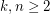, the 2-stage Shuffle-Exchange graph/network, denoted, is the simple-regular bipartite graph with the ordered pair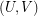of linearly labeled parts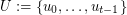and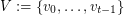, where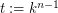, such that verticesand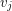are adjacent if and only if(see Fig.1).

Given integers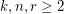, the-stage Shuffle-Exchange graph/network, denoted, is the proper (i.e., respecting all the orders) concatenation ofidentical copies of(see Fig.1).

Letbe the smallest integer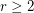such that the graphis rearrangeable.

Problem   Find.
Conjecture.

A mask for the graphis a-regular bipartite multigraph with the bipartition. The graphis said to be rearrangeable if for every its mask there exists a collection, called routing, of corresponding mutually edge-disjoint paths inconnecting its end parts. (For simplicity, we do not provide here a more general definition for rearrangeability of graphs.)

Note thatis a simple-partite graph with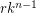vertices andedges, and any route for it consists exactly of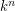paths. Also,is equivalent to rearrangeability of.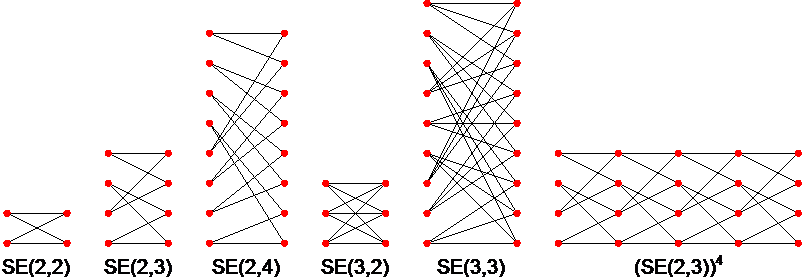Figure 1. Examples of multistage Shuffle-Exchange graphs.

For example, according to the conjecture, the graph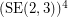(see Fig. 1) is rearrangeable, which is a well known result.

The problem and conjecture are equivalent "graph-theoretic" forms of remarkable Shuffle-Exchange (SE) problem and conjecture due to the following identity (that is not hard to show by normal reasoning):

Theorem.

The definition ofand more on SE problem/conjecture including the other 2 main forms of them, combinatorial and group-theoretic, and a survey of results can be found here.

## Bibliography

*[S71] H.S. Stone, Parallel processing with the perfect shuffle, IEEE Trans. on Computers C-20 (1971), 153-161.

*[B75] V.E. Beneš, Proving the rearrangeability of connecting networks by group calculation, Bell Syst. Tech. J. 54 (1975), 421-434.

* indicates original appearance(s) of problem.